Logistic Distribution

#Logistic Distribution

The most common use of the Logistic Distribution is in describing growth. As a result, it is popularly used for economic demographic purposes. It can also be used as subsititute for the normal distribution under certain circumstances.

The Logistic Distribution is defined for all values of x, positive and negative. It has two parameters, a and b. The first parameter, a, must be positive, but the second parameter, b, can have any value. The distribution is: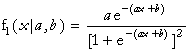where a>0. (x and b can have any value.)

The equations for the mean and variance are: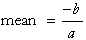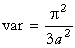The equations for the parameters are: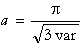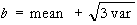Versions: DPL Professional, DPL Enterprise, DPL Portfolio